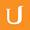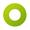## 01-22 Probability Review Pt 3 Solution

• 0:00 - 0:04
We can compute this using the formula for conditional probability.
• 0:04 - 0:09
In this case what A is is the probability the coin toss is valid.
• 0:09 - 0:18
A is valid, and we know that the probability of A is equal to 0.99998.
• 0:18 - 0:21
What B is is the probability that it's heads.
• 0:21 - 0:29
So the probability of B is the probability of H, which is 0.49999.
• 0:29 - 0:32
Now we just have to plug these into the formula.
• 0:32 - 0:36
But what we need to use the formula is the probability of A intersect B.
• 0:36 - 0:42
And what A is the probability of A intersect B. What A is is valid.
• 0:42 - 0:46
• 0:46 - 0:52
• 0:52 - 0:57
We know the probability of tails is 0.49999.
• 0:57 - 1:04
That means using the formula we have the probability of tails, which is 0.49999,
• 1:04 - 1:07
which is the probability of A intersect B.
• 1:07 - 1:12
We're dividing that by the probability of A,
• 1:12 - 1:18
which is a valid event which is 0.99998.
• 1:18 - 1:20
We get 0.5.
• 1:20 - 1:23
I should note that this is not the case for real coin tosses.
• 1:23 - 1:28
There is no physical coin ever manufactured that has exactly
• 1:28 - 1:30
chances of landing on both sides.
• 1:30 - 1:34
In fact, with real coin tosses, at least with American currency,
• 1:34 - 1:39
there is a much higher percentage--much higher meaning close to 51%
• 1:39 - 1:43
rather than 50%--that the coin lands on the same side that it started on.
• 1:43 - 1:46
When we talk about mathematical coin tosses,
• 1:46 - 1:48
we're going to assume that there is no edge case
• 1:48 - 1:50
and that it's equally likely
• 1:50 - 1:53
that we have a uniform distribution and there are only 2 outcomes.
• 1:53 - 1:56
When we talk about mathematical coin tosses,
• 1:56 - 1:58
we're going to assume that we have a uniform distribution,
• 1:58 -
and there are only two outcomes.
Title:
01-22 Probability Review Pt 3 Solution
Description:

more » « less
Team:Udacity
Project:
CS387 - Applied Cryptography
Duration:
02:01Amara Bot added a translation

• Amara Bot